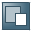Show TOC

###Interest Calculations in Dunning SystemUse the Dunning Terms - Setup window to determine how interest is calculated. The following examples demonstrate each method.

#### Prerequisites

1. There are 30 days in each month.

2. There are 360 days in a year.

#### Example

Calculate Interest Value on Remaining Sum:

Due Date

Document Type

Total

01.01.03

Invoice

10000

01.02.03

Payment

3000

Remaining Sum is 10000-3000 =

7000

Interest rate is 7% and the dunning wizard is processed on 01.03.03

7000X7%X60*/360 = 81.6667

* number of days counted since the due date of the invoice and the date in which the dunning wizard is processed.

Calculate Interest Value on Original Sum:

Due Date

Document Type

Total

01.01.03

Invoice

10000

01.02.03

Payment

3000

Interest rate is 7 % and the dunning wizard is processed on the 1.3.03

(10000X7%X30/360) + (7000X7%X30/360) = 99.16666

The value of the first parenthesis is the interest that was accumulated until the first payment was created.

The value in the second parenthesis is the interest accumulated until the wizard was processed.